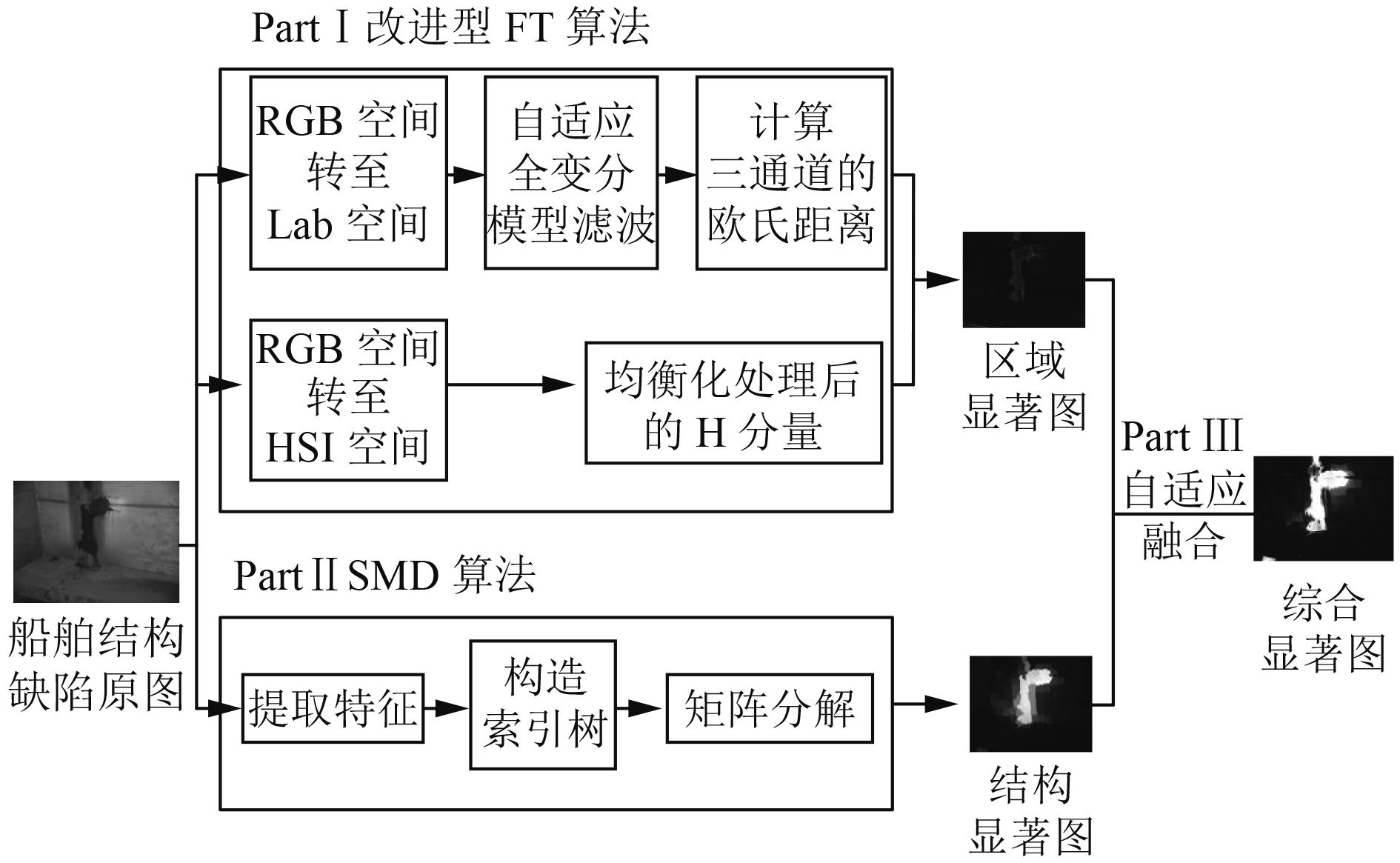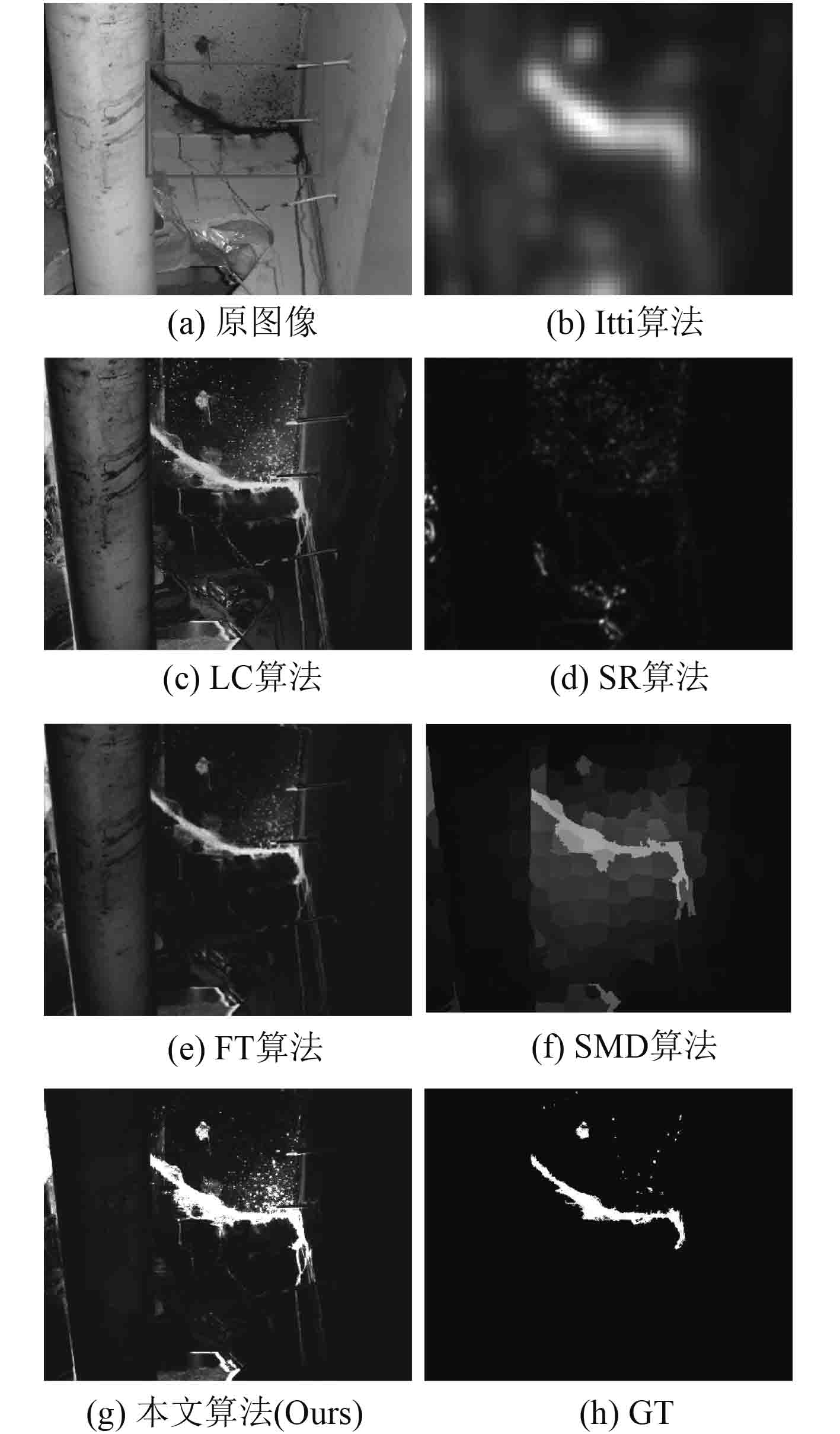﻿ 基于视觉显著性的船舶结构缺陷检测研究
 舰船科学技术2019, Vol. 41Issue (6): 53-56PDF

Ship structure defect detection based on visual saliency
CHEN Shu-yue, SHEN Xin-xia, LU Gui-rong
School of Information Science and Engineering, Changzhou University, Changzhou 213164, China
Abstract: Aiming at the problem that the background structure of ship structure defects is complex and the defects are difficult to detect, a defect detection algorithm based on visual saliency is proposed. The improved FT algorithm replaces the Gaussian filter with an adaptive total variation model, incorporates the H component, and enhances the smoothness of the defect image background and the expression ability of the defect local color contrast. The SMD algorithm is used to capture the image structure information, and the image feature matrix is decomposed to highlight the difference between the ship defect and the background. The explicit map generated by the improved FT algorithm and the SMD algorithm is merged into a comprehensive saliency map by an adaptive fusion algorithm. By comparing the ship's defects, the results show that the proposed algorithm can effectively detect the ship defect area and significantly improve the defect detection precision.
Key words: ship defect detection     adaptive total variation model     frequency tuning algorithm(FT)     structure matrix decomposition algorithm(SMD)     visual saliency
0 引　言

1 船舶结构缺陷检测算法图 1 船舶结构缺陷检测算法流程框图 Fig. 1 Ship structure defect detection algorithm flow chart
1.1 基于改进型FT算法的区域显著性算法

 ${{S}_{0}}\left( i,j \right)=\left\| {{ u}_{\mu }}-{{u}_{\omega hc}}\left( i,j \right) \right\|\text{。}$ (1)

FT算法理论简单，检测效果良好，将其应用于船舶结构缺陷检测中，存在2点不足之处：根据人工经验选取的高斯滤波器的模板尺度较单一；FT算法要求缺陷与背景区域存在一定的对比性。针对不足之处，对FT算法进行改进如下：

1）对于高斯滤波器模板尺寸选取问题，提出采用自适应全变分模型替代高斯滤波器。该模型根据图像边缘或纹理信息丰富的区域的梯度值高于平坦区域的特性，对图像分块处理，将船舶结构缺陷图像划分为边缘区域和平坦区域，并自适应选择去噪模型。对于边缘区域，使用边缘性较强的L1范数的各向异性的全变分模型，反之，使用L2范数的各向同性的全变分模型。该模型的增广拉格朗日函数为：

 $\begin{split}{{L}_{A}}\left( {{w}_{ij}},u,\lambda \right)=&\sum\limits_{i,j=1}^{n}{\left\| {{w}_{ij}} \right\|+\sum\limits_{i,j=1}^{n}{\frac{\beta }{2}\left\| {{D}_{ij}}u-{{w}_{ij}} \right\|_{2}^{2}}}+\\ &\frac{\lambda }{2}\left\| u-f \right\|_{2}^{2}\text{。} \end{split}$ (2)

 $\begin{split} w_{ij}^{k+1}=& {\rm {shrinkage}}\left( {{D}_{ij}}{{u}^{k}},\beta \right)=\\ &\max \left\{ \left\| {{D}_{ij}}{{u}^{k}} \right\|-\frac{1}{\beta },0 \right\}\times \frac{{{D}_{ij}}{{u}^{k}}}{\left\| {{D}_{ij}}{{u}^{k}} \right\|}\text{。} \end{split}$ (3)

 $\left( \sum\limits_{i,j}{D_{ij}^{T}{{D}_{ij}}}+\frac{\lambda }{\beta }{{K}^{\rm {T}}}K \right){{u}^{k}}=\sum\limits_{i,j}{D_{ij}^{T}w_{ij}^{k+1}}+\frac{\lambda }{\beta }{{K}^{\rm {T}}}f\text{。}$ (4)

2）针对FT算法对亮度低及背景颜色差异较小的缺陷区域不敏感的问题，将HSI颜色空间中H分量融入船舶结构缺陷特征表达，增强图像局部颜色的对比度，便于捕获船舶结构缺陷区域与背景之间的细微差异。融合式为：

 ${{S}_{1}}\left( i,j \right)=\sqrt{H\left( i,j \right){{S}_{0}}\left( i,j \right)}\text{。}$ (5)

1.2 结构显著性算法

 $\mathop {\min }\limits_{L,M} \psi \left( { L} \right) + \alpha \varOmega \left( { M} \right) + \mu \Theta \left( {{ L},{ M}} \right)\begin{array}{*{20}{c}} {}\!\!&\!\!{{\rm s}.{\rm t}.}\!\!&\!\!{{ N} = { L} + { M}}\text{，}\!\!\!\!\! \end{array}$ (6)
 $\psi \left( { L} \right) = rank\left( { L} \right) = {\left\| { L} \right\|_ * } + \varepsilon\text{，} \hspace{95pt}$ (7)
 $\varOmega \left( { M} \right) = \sum\limits_{i = 1}^d {\sum\limits_{j = 1}^{{n_i}} {\nu _j^i} } {\left\| {{{ M}_{G_j^i}}} \right\|_p}\text{。} \hspace{95pt}$ (8)

 $\Theta \left( {{ L},{ M}} \right) = \frac{1}{2}\sum\limits_{i,j = 1}^K {\left\| {{{ M}_i} - {{ M}_j}} \right\|} _2^2{n_{i,j}}\text{。}$ (9)

 $Sal\left( {{P_i}} \right) = {\left\| {{{ M}_i}} \right\|_1}\text{。}$ (10)

1.3 自适应融合算法

 ${S_3}\left( {i,j} \right) = {\omega _1}{S_1}\left( {i,j} \right) + \left( {1 - {\omega _1}} \right){S_2}\left( {i,j} \right)\text{，}$ (11)
 ${\omega _1} = \frac{{\sum\limits_{i,j} {{S_2}\left( {i,j} \right)} }}{{\sum\limits_{i,j} {{S_1}\left( {i,j} \right)} + \sum\limits_{i,j} {{S_1}\left( {i,j} \right)} }}\text{。} \hspace{42pt}$ (12)

2 实验结果与分析

2.1 船舶结构缺陷检测对比实验图 2 不同显著性算法检测结果对比 Fig. 2 Comparison of test results of different saliency algorithms
2.2 算法检测性能评价

 $P = \frac{{TP}}{{TP + FP}},R = \frac{{TP}}{{TP + FN}},F = \frac{{\left( {1 + {\beta ^2}} \right) \times P \times R}}{{{\beta ^2} \times P + R}}\text{。}$ (13)表 1 使用不同算法进行缺陷检测的结果比较 Tab.1 Comparison of results using different algorithms for defect detection
3 结　语

  李瑛. 船体表面缺陷检测系统研究[J]. 舰船科学技术, 2016(24): 109-111. LI Ying. Research on hull surface defect detection system[J]. Ship Science and Technology, 2016(24): 109-111.  OULLETTE R, BROWNE M, HIRASAWA K. Genetic algorithm optimization of a convolutional neural network for autonomous crack detection[M]. Evolutionary Computation, 2004: 516–521.  EICH M, BONNINPASCUAL F, GARCIAFIDALGO E, et al. A robot application to marine vessel inspection[J]. Journal of Field Robotics, 2014, 31(2): 319-341. DOI:10.1002/rob.2014.31.issue-2  BONNíN-PASCUAL F, ORTIZ A. Detection of Cracks and Corrosion for Automated Vessels Visual Inspection[J]. Integrated Pest Management Reviews, 2010, 3(2): 111-120.  BONNIN-PASCUAL F, ORTIZ A. Corrosion Detection for Automated Visual Inspection[M]. Austria: In Tech, 2014: 619–632.  ACHANTA R, HEMAMI S, ESTRADA F, et al. Frequency-tuned salient region detection[C]. Conference on Computer Vision and Parttern recognition, 2009: 1597–1604.  PAN Jinquan, CHEN Shuner, FENG Yuanhua, et. al. Method of Image Block Denoising Based on Adaptive Total Variation[J]. International Journal of Computer Techniques, 2016, 4(3): 174-179.  PENG H, LI B, LING H, et al. Salient Object Detection via Structured Matrix Decomposition[J]. IEEE Transactions on Pattern Analysis & Machine Intelligence, 2017, 39(4): 818-832.  ITTI L, KOCH C. Computational modelling of visual attention[J]. Nature Reviews Neuroscience, 2001, 2(3): 194-203. DOI:10.1038/35058500  ZHAI Y, SHAH M. Visual Attention detection in video sequences using spatiotemporal cues[C]. New York: Proceedings of the14th Annual ACM International Conference on Multimedia, 2006: 815–824.  HOU X, ZHANG L. Saliency Detection: A spectral residual approach[C]. Minneapolis: 2007 IEEE Computer Society Conference on Computer Vision and Pattern Recognition (CVPR 2007), 2007: 1–8.